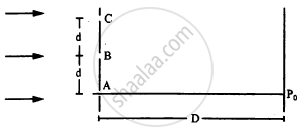Department of Pre-University Education, KarnatakaPUC Karnataka Science Class 12
Advertisement Remove all ads

# The Following Figure Shows Three Equidistant Slits Being Illuminated by a Monochromatic Parallel Beam of Light. - Physics

Sum

The following figure shows three equidistant slits being illuminated by a monochromatic parallel beam of light. Let $B P_0 - A P_0 = \lambda/3\text{ and }D > > \lambda.$ (a) Show that in this case $d = \sqrt{2\lambda D/3}.$ (b) Show that the intensity at P0 is three times the intensity due to any of the three slits individually.Advertisement Remove all ads

#### Solution

(a) Given:-

Wavelength of light = $\lambda$

Path difference of wave fronts reaching from A and B is given by

$∆ x_B = {BP}_0 - {AP}_0 = \frac{\lambda}{3}$

$\Rightarrow \sqrt{D^2 + d^2} - D = \frac{\lambda}{3}$

$\Rightarrow D^2 + d^2 = D^2 + \frac{\lambda^2}{9} + \frac{2\lambda D}{3}$

We will neglect the term $\frac{\lambda^2}{9},$ as it has a very small value.

$\therefore d = \sqrt{\frac{\left( 2\lambda D \right)}{3}}$

(b) To calculating the intensity at P0consider the interference of light waves coming from all the three slits.

Path difference of the wave fronts reaching from A and C is given by

${CP}_0 - {AP}_0 = \sqrt{D^2 + \left( 2d \right)^2} - D$

$= \sqrt{D^2 + \frac{8\lambda D}{3}} - D ........\left(\text{Using the value of d from part a} \right)$

$= D \left\{ 1 + \frac{8\lambda}{3D} \right\}^\frac{1}{2} - D$

Expanding the value using binomial theorem and neglectingthe higher order terms, we get

$D\left\{ 1 + \frac{1}{2} \times \frac{8\lambda}{3D} + . . . \right\} - D$

${CP}_0 - {AP}_0 = \frac{4\lambda}{3}$

So, the corresponding phase difference between the wave fronts from A and C is given by

$\phi_c = \frac{2\pi ∆ x_C}{\lambda} = \frac{2\pi \times 4\lambda}{3\lambda}$

$\Rightarrow \phi_c = \frac{8\pi}{3}\text{ or }\left( 2\pi + \frac{2\pi}{3} \right)$

$\Rightarrow \phi_c = \frac{2\pi}{3}...........(1)$

Again, $\phi_B = \frac{2\pi ∆ x_B}{\lambda}$

$\Rightarrow \phi_B = \frac{2\pi\lambda}{3\lambda} = \frac{2\pi}{3}..........(2)$

So, it can be said that light from B and C are in the same phase, as they have the same phase difference with respect to A.

Amplitude of wave reaching P0 is given by

$A = \sqrt{\left( 2a \right)^2 + a^2 + 2a \times a\cos\left( \frac{2\pi}{3} \right)}$

$= \sqrt{4 a^2 + a^2 + 2 a^2 \sqrt{3}}$

$\therefore l_{po} = K \left( \sqrt{3} r \right)^2 = 3 K r^2 = 3l$

Here, I is the intensity due to the individual slits and Ipo is the total intensity at P0.

Thus, the resulting amplitude is three times the intensity due to the individual slits.Concept: Refraction of Monochromatic Light
Is there an error in this question or solution?
Advertisement Remove all ads

#### APPEARS IN

HC Verma Class 11, 12 Concepts of Physics 1
Chapter 17 Light Waves
Q 28 | Page 382
Advertisement Remove all ads
Advertisement Remove all ads
Share
Notifications

View all notifications

Forgot password?